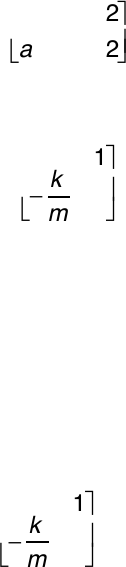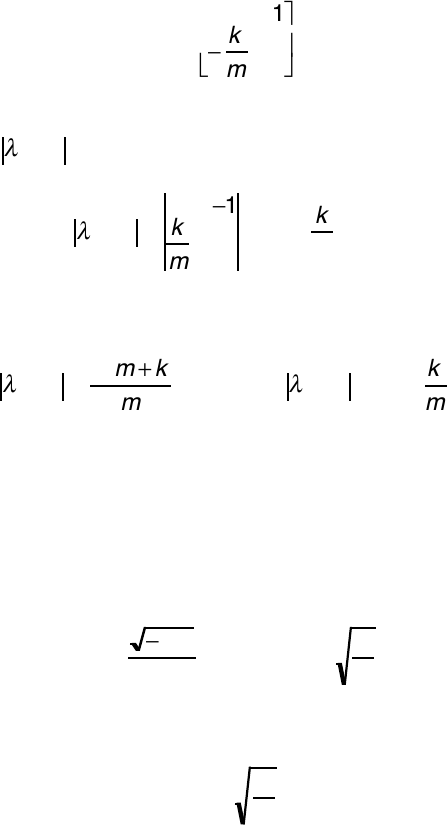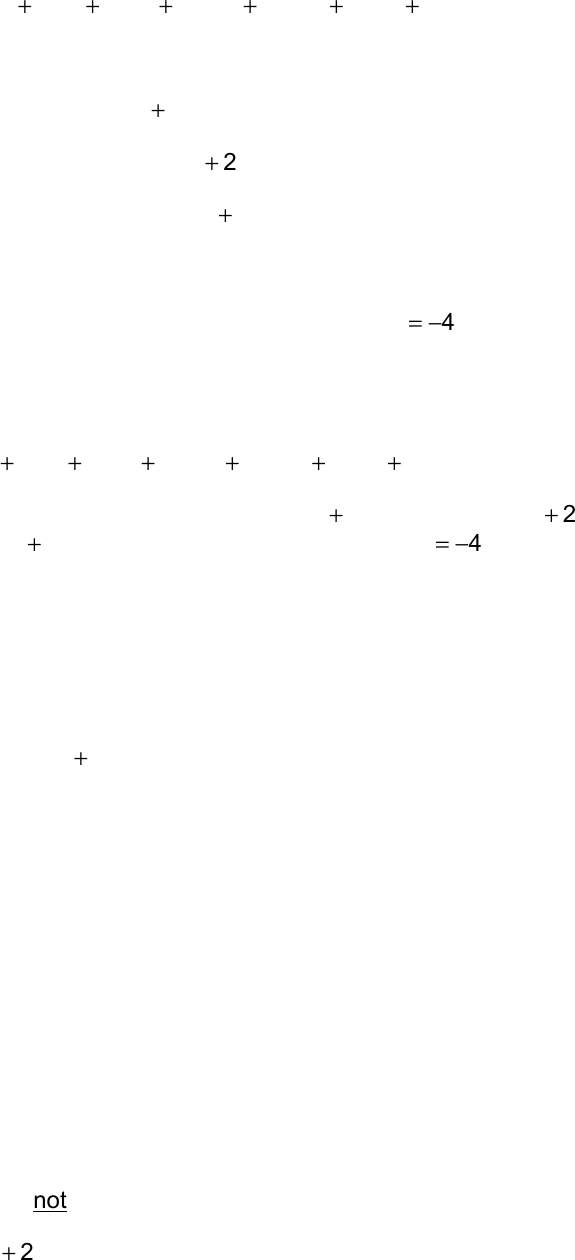Textbook Notes (290,000)
US (110,000)
LSU (20,000)
ME (300)
All (5)
Chapter

# HW 2 SolutionExam

Department
Mechanical Engineering
Course Code
ME 3143
Professor
All

This preview shows pages 1-3. to view the full 19 pages of the document.ME 3143 F’11 Problem Set 2 Solution
1
ME 3143
Fall 2011
Problem Set 2 Solution
1. Maple is a package for doing symbolic (and numerical) mathematics.
In this class we will use it for some linear algebra with symbolic state
matrices.
Open a window in Maple. Type the following command to load the
linear algebra library:
with(linalg); End all commands with a semicolon
This gives access to all linear algebra functions. A matrix, A, is defined
using the following syntax:
A := matrix(2,2,[a11,a12,a21,a22]);
Yielding (if successful):
A:=
a11 a12
a21 a22
!
"
#
\$
%
&
(a) Enter the matrix:
A:=
0 1
!k
m
0
"
#
\$
%
&
'
(b) Evaluate the characteristic polynomial of the matrix.
Compare this to the result obtained with the command
charpoly(A,lambda).
(c) Use the eigenvals(A) command to evaluate the
eigenvalues of the matrix. Check your result by hand.
Given: A state matrix:
A:=
0 1
!k
m
0
"
#
\$
%
&
'
.
Find: Use Maple and hand calculations to (a) evaluate the
characteristic polynomial and (b) the eigenvalues for the system
represented by the state matrix.

Only pages 1-3 are available for preview. Some parts have been intentionally blurred.ME 3143 F’11 Problem Set 2 Solution
2
Solution: (a) Enter the matrix. The result is:
A:=
0 1
!k
m
0
"
#
\$
%
&
'
(b) Evaluate the characteristic polynomial for the state matrix, by
evaluating
!
I"A
:
!
I"A=
!
"1
k
m
!
=
!
2+k
m
=0
The charpoly command yields:
!
I"A=
!
2m+k
m
=0#
!
I"A=
!
2+k
m
Which is the same as obtained by hand, after a little reduction.
Maple results often require some manipulation to get to the final
result.
(c) Next evaluate the eigenvalues and the roots of the characteristic
equation. Using the eigenvals(A) command gives:
Solve for the roots of the characteristic polynomial:
!
= ± jk
m
Both show that the system has a pair of complex conjugate
eigenvalues that are equal to the roots of the characteristic
polynomial. We will learn later that the complex conjugate roots
indicate oscillatory system response.
2. We will also use Matlab™ and Simulink™ extensively this semester. One
use for Matlab™ is the factoring and multiplication of polynomials.
Polynomials are represented by a vector containing the coefficients:
s4+3s2+12s+5!1 0 3 12 5
[ ]
Several commands are useful for manipulating polynomials, including:
roots, conv, deconv, polyval.

Only pages 1-3 are available for preview. Some parts have been intentionally blurred.ME 3143 F’11 Problem Set 2 Solution
3
!
!+""
!
#+#\$
!
\$+"!%
!
&+'%%
!
'+'&\$
!
+"'(
Use these four commands on the above polynomial.
(a) For conv multiply by
!
+!
.
(b) Use deconv to divide out
!
+!
,
(c) Use deconv to divide out
!
+!
. Is there any difference in the
result from (b)? Explain. (HINT: Use roots to help formulate
(d) Use polyval to evaluate the polynomial at
!
=!!
. Check the
polyval result by hand.
Given: A polynomial:
!
!+""
!
#+#\$
!
\$+"!%
!
&+'%%
!
'+'&\$
!
+"'(
Find: Use Matlab to: (a) multiply by
!
+!
, (b) divide by
!
+!
,
(c) Divide by
!
+!
; (d) Evaluate the polynomial at
!
=!!
.
Solution: The polynomial is represented as a vector of its
coefficients in Matlab:
!!
( )
=
!
!+""
!
#+#\$
!
\$+"!%
!
&+'%%
!
'+'&\$
!
+"'( !
!
=" "" #\$ "!% '%% '&\$ "'(
"
#
\$%
&
'
(a) The factor
!
+!
is also represented as a vector:
!!
( )
=
!
+!!
!
=" !
"
#
\$%
&
'
Multiply polynomials using the conv function:
!!
( )
=
!!
( )
!!
( )
=
!
+!
( )
!
"+##
!
\$+\$%
!
%+#"&
!
!+'&&
!
'+'!%
!
+#'(
( )
=)*+,
!
-
"
( )
Evaluation yields:
!!
( )
=! !" #\$ %&' \$\$# !()* #&& %)(
!
"
#\$
%
&=
!
\$+!"
!
)+#\$
!
*+%&'
!
"+\$\$#
!
%+!()*
!
&+#&&
!
+%)(
( )
This function is not order dependent.
(b) The factor
!
+!
is the vector: